Estimating the Investment Value of an Assetandrsquo;s Liquidity | OMICS International

# Estimating the Investment Value of an Asset’s Liquidity

Yuriy V Kozyr*

Department of Theoretical Economy and Mathematic Research, Central Economics and Mathematics Institute of Russian Academy of Sciences, Moscow, 117418, Russia

*Corresponding Author:
Dr. Yuriy V. Kozyr
Department of Theoretical Economy and Mathematic Research
Central Economics and Mathematics Institute of Russian Academy of Sciences
Moscow, 117418, Russia
Tel: +7(495) 924-3276
Fax: +7(495) 779-1324
E-mail: [email protected]

Received date: February 25, 2014; Accepted: September 29, 2014; Published date: October 10, 2014

Citation: Kozyr YV (2014) Estimating the Investment Value of an Asset’s Liquidity. Bus Eco J 5:109. doi: 10.4172/2151-6219.1000109

Copyright: © 2014 Kozyr YV. This is an open-access article distributed under the terms of the Creative Commons Attribution License, which permits unrestricted use, distribution, and reproduction in any medium, provided the original author and source are credited.

Visit for more related articles at Business and Economics Journal

#### Abstract

This article presents the solution to the problem of the investment choice between cash funds and earning assets (the latter is associated with bonds) and describes the situation-related approach to estimating both the relative and absolute values of liquidity.

#### Keywords

Value of liquidity; Absolute liquidity; Relative liquidity; General liquidity; Additional liquidity; Liquidity costs; Utility function; Equalizing interest rate; Certainty cash equivalent

#### Introduction

In their book “Principles of Corporate Finance”, the authors Richard A. Brealey and Stewart C. Myers address the problem of estimating the value of liquidity. The authors raise the following question: how should the company, with the other conditions being equal, allocate their investments between more and less liquid assets. They find this question difficult to answer. Undoubtedly, every company should be ready to raise money quickly, but there is no good theory that explains what the sufficient level of cash funds is and how fast a company must find this money .

Thus, the authors emphasize the fact that at least by the time the aforementioned book was written, the academic community had lacked approaches to estimating the value of liquidity (Let’s define liquidity as the asset’s ability (probability) to convert into another asset (to sell) both quickly and without significant financial losses; and by liquidity value we shall mean the additional value of the asset (property, goods, services), which is conditioned by its higher liquidity compared with another asset). The existing “topic-related” models include only cash balance models; e.g., the Miller-Orr Model , which enables one to decide on changes in the proportions of the portfolio consisting of cash and shares. Nevertheless, one should realize that these models don’t allow the value of liquidity itself to be estimated. Taking all of this into account, an attempt was made to decrease the number of the unsolved financial theoretical problems (As according to the author’s opinion, one out of ten unsolved problems is half-solved in this paper) by five percent. And here are the results achieved in the course of the experiment.

We started with the following variables of the hypothesized (i.e., yet unfound) liquidity function including asset quote spread, fixed conversion costs, interest rate on securities, alternative rate of interest, and time required for conversion of one asset into another. In some cases several assumptions were made about the expected reality; for instance, the introduction of such parameters as expected time for holding an asset in cash before being converted into another asset; probability of occurrence for the need to convert for a fixed time; probability of sale for an asset (i.e., probability for a less liquid asset to convert into another one of higher liquidity) for a fixed time without changes in its price quoted earlier.

The obtained results made it possible for every particular case to determine (with due regard for a known set of data) whether it is more profitable to hold assets either in cash or a non-cash form (Concerning the decision on the aforementioned preferable choice, the method given hereinafter can be somewhat an alternative to the Miller-Orr model. Nevertheless, as opposed to this model, the proposed method is not for the optimization of the investment portfolio containing cash and non-cash assets). Unfortunately, the problem of optimal asset allocation between cash and non-cash forms is still unsolved, being still oriented in its solution to the Miller-Orr model and other similar techniques.

Let us proceed to give the proposed method for estimating the value of liquidity.

#### Criteria of Alternative Liquidity

Simulate the situation that the company’s top managers face a choice whether to place temporarily surplus funds in alternative investments (deposit, bonds, other companies’ shares), or to reserve these funds for some unforeseen situations, or somehow to allocate them between the first two variants. The first possibility (i.e., to place surplus funds in alternative investments) is advantageous in providing the highest interest from using this funds. The weakness to this approach (at the same time, being the strength to the second approach) deals, firstly, with the risk of non-repayment within a scheduled period; secondly, with the non-vanishing probability of occurrence for the need to use these funds before a scheduled period (In the virtue of unforeseen circumstances related to the necessity of a stable performance of a company’s main business); thirdly, with the fact that the conversion of these funds into other assets involves both time losses and monetary expenses. In order to compare the alternatives of holding either non-interest cash reserve or non-cash assets generating interests, it seems reasonable to lower the interest rate of a non-cash asset, thus showing the factor of lower (As compared with a cash flow) liquidity for this non-cash asset. Let us discuss the criteria (One more criterion is given in Part 2 of this paper) given hereinafter, which represent the mechanism of this adjustment and simultaneously the criteria of relevant decisions.

Criterion 1: If the following inequality holds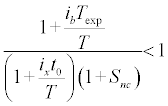(1)

These assets would be preferably held in cash rather than bonds, or vice versa for the contrary case.

Inequality (1) contains the following elements:

Texp – expected time for holding assets in cash before being converted into project X, year fraction;

t0 – minimum time required for bonds to be converted (in this case) into cash, year fraction;

Snc – spread transaction costs of converting bonds (in general case, non-cash assets) into cash, % or unit fraction;

ix – interest rate of return on project X, % or unit fraction;

ib – interest rate of return on bonds (in general case, non-cash assets), % or unit fraction,

T – period of time related to the rates of return ib and ix; e.g., if the rate of return is given in p.c.p.a., then T is equal either to one year as soon as t0 and Texp are expressed in year fractions, or to 365 (243) as soon as ib, ix, Texp, and t0 are expressed in days.

The term “project X” is referred to as any possible alternative to bonds such as settlements with creditors and suppliers for a further successful performance of the main business, the return on which equals ix%; and the rate of interest on this alternative is expected to be higher than that on bonds.

Criterion 1 makes it possible to obtain the condition of feasibility for cash to be converted into a non-cash asset. Such feasibility takes place when the following equivalent conditions are observed: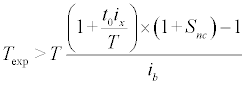(2)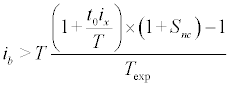(3)

All the notions correspond to those accepted in (1).

Criterion 2: (stronger as compared with Criterion 1).

If the value of the utility function for cash funds (Utility function is referred to as the function, which reflects the value adjusted for a degree of the interest rate liquidity and enables to compare the interest rates of the assets of different liquidity) Wc is higher than the value of the utility function for a bond Wb, then, again, the assets would be better to hold in cash, and vice versa.

In this case: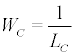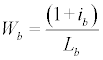(4)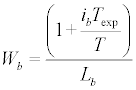(5)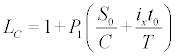(5a)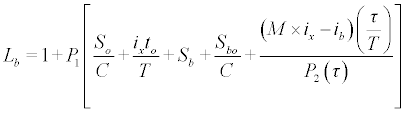(6)

Or more precisely: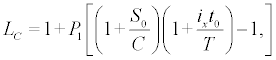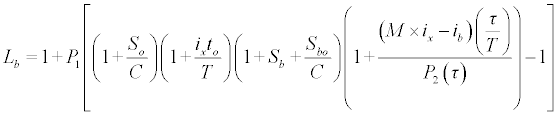(7)

Where:

Lc - cash liquidity costs, unit fraction;

Lb - bond liquidity costs, unit fraction;

Sb – bond quote spread (a non-cash asset, in general case), unit fraction;

Sb0 - fixed costs of selling bonds (a non-cash asset, in general case), RUB;

S0 - fixed costs of investing money into project X, RUB;

C – absolute value of the size of conversion-involved assets, RUB;

S0/C –constant of investment costs for cash funds (C) expressed in a relative form, unit fraction;

Sb0/C – constant of the costs of selling securities (a non-cash asset, in general case) for a sum of C, expressed in a relative form, unit fraction;

t0 – time required for cash conversion into project X;

τ- average time for bond sales (a non-cash asset, in general case) from the moment of price quotation or an ask quote till the moment of a bid quote (or an actual transaction);

T – period of time related to the rates of return; e.g., if the rates of return are given in p.c.p.a., then T is equal either to one year as soon as τ and t0 are expressed in year fractions, or to 365 as soon as ib, ix, τ and t0 are expressed in days;

M – scale/measuring factor that reflects the loss build up rate for the time of selling a non-cash asset (τ or (τ + t0)). This means that if the conversion of a non-cash asset (priced C, generating return ib) into cash funds is delayed, the losses of the main business possessing profitability ix equal MC.

P1 – probability of occurrence for the need to convert for a calculated interval of time equal to the expected time of holding an asset Texp in the appropriate form, 0≤P1≤1;

P2(τ) – probability of selling bonds (a non-cash asset, in general case) for the time τ without lowering the existing quotes, τ ≥ t0, 0 < P2 ≤ 1.

As soon as it is impossible to avoid subjectivism when estimating the values P1 and P2, it is reasonable to begin with substituting the values {0, 1} (note that as a minimum permissible value for P2, one would select a nonzero value), obtaining as reference values three possible boundary values for Wb (Three and not four values because if P1 = 0, the function Wb will take the same values whatever the value of P2) and two for Wc, and further proceeding with substituting any other intermediate value corrected with due regard for objective and subjective (statistical or expert) estimates.

Note 1: Another name (similar in its content) for utility function in this case can be the concept “relative investment value”. According to IVS-2011 (International Valuation Standards, 2011), “investment value is the value of an asset to the owner or a prospective owner for individual investments or operational objectives. This is an entity-specific basis of value…Investment value reflects the circumstances and financial objectives of the entity for which the valuation is being produced. It is often used for measuring investment performance.”

Note 2: The difference between expressions (5) and (5a) is reasoned by (5) taking a general form and (5a) being a calculation formula.

Note 3: If P1=1, the denominators in (6) and (7) represent a measure of liquidity (or that of illiquidity); and if P1=0, the denominator is always equal to one. Furthermore, it is obvious that the asset itself doesn’t become more liquid, but the utility of holding this asset will equal the utility of holding cash (certainly, under equality conditions for the numerator, i.e., in the absence of interests generated by this asset), which is reflected by the utility function.

It is also essential to point out the interrelation between Snc in (1) and both Sb and Sb0/C in (6) and (7):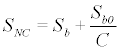(8)

It is worthy of special notice that in expressions (6)-(7), C is associated with the size of assets (cash or securities), which are incommensurately smaller than the market capacity of a corresponding asset, thus provoking both the decrease in the fixed transaction cost ratio if C becomes higher, and a consequent increase in liquidity. If this condition isn’t satisfied, the aforementioned model fails to work because if the size of C is commensurate with the capacity of the whole market, the operator pressures the market, thus reducing the liquidity of this asset. To estimate liquidity in these cases, one should apply models, which are different from the previously described one and which takes into account the factor of an asset’s pressure on the market.

#### Value of Liquidity

Being applied, Criterion 2 makes it possible to estimate the values of liquidity, illiquidity or an illiquid asset. This can be executed with the method similar to the certainty equivalent method used in the capital asset pricing model. Let us give several examples.

Example 1: Assume that the calculations on Criterion 2 (the results calculated with formulas (4) – (7)) gave the following results for the functions of money and bond utilities: Wc = 0.99, Wb = 0.94. Suppose that the rate of interest ib on bonds is 12%. We denote cash liquidity costs (Liquidity costs of cash funds are referred to as constants, e.g., bank commission when transferring sums from one account to another, expressed in a relative form, and variable costs related to the loss of profit due to the lost time spent on converting cash funds in a better alternative asset. For example, for the metallurgic plant it is more important to possess non-cash assets in the form of ore or coke reserves rather than cash reserve, because if coke or ore are cash-purchased, this means that the main funds are converted into ore and coke, whilst the inverted conversion “coke into cash funds” doesn’t take place. In this situation, the liquidity costs for cash funds Lc are higher than those for non-cash assets Lb) by Lc. Then the substitution of the initial data into formulas (4)-(5) will result in the following expressions: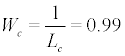(9)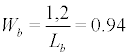(10)

As Wc>Wb and the numerator Wb is 12% higher than the numerator Wc, consider the following two questions: 1) At what interest rate on a bond does the utility function for a bond equal the utility function for cash funds? 2) At what bond liquidity costs does the utility function for a bond equal the utility function for cash funds? The last question represents only a theoretical rather than practical concern because, firstly, it is clearly that a bond’s liquidity will never surpass the liquidity of cash; secondly, the objectives of the study don’t involve the consideration of liquidity costs as variable parameters. Therefore, let us keep our attention focused on asking the first question. To meet the condition formulated in the first question, the following equality should hold: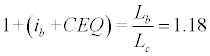(11)

Where:

CEQ – certainty equivalent of a cash flow, i.e., the interest rate, which lessens the gap between the utility of holding money and that of holding a non-cash asset equivalent to a market price with zero yields (ib);

(ib + CEQ) – interest rate, which equalizes the utility of holding a non-cash asset (bonds) with that of holding money.

If ib = 0, the equalizing interest rate equals the certainty equivalent (CEQ).

From (11), we calculate the values of both equalizing rate and certainty equivalent: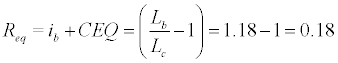(12)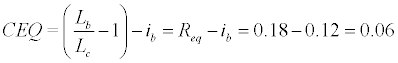(13)

Where:

Req – equalizing interest rate.

Thus, for the conditions of our example we found the equalizing interest rate = 18% and the certainty equivalent = 6% (in this case, annualized values are given). This means the following: until the interest rate on bonds is lower than the equalizing rate (18%), the conversion of money into bonds would be ineffective under the existing conditions.

Study another example.

Example 2: Let Wc=0.99, Wb=1.05, ib=12%. In this case the benefit from the interest rate on bonds obviously exceeds their additional losses of liquidity as compared with cash funds. Therefore, it would be worth examining another question: to what level can the rate ib decrease so that the equality holds Wc=Wb?

Solving equation (12) for this example gives the answer to this question, calculating the equalizing interest rate as Wc X(1+ ib)/ Wb-1=5.6%. Hence the value of the certainty equivalent for money is calculated as CEQ = 5.6% -12% = -6.4%, which determines the level of dominance for the utility of bonds due to their interest rate over the utility of money due to their higher liquidity. In this case, the CEQ represents a so-called “relative safety margin” for the utility of holding bonds:

• Negative CEQ value shows a higher utility of a non-cash asset as compared with cash funds;

• The modulus of the CEQ value (safety margin) shows how much the interest rate on bonds can decrease from the current level so that the equivalence of investments (expressed as their utility functions) in cash funds and bonds executes.

For the conditions of the aforementioned example, the safety margin had a positive value (6%), which proved the contrary – to achieve the efficiency of holding bonds, which equals the efficiency of cash funds, the interest rate on bonds should go up by 6%.

Study the interrelations between the investment values for liquid and illiquid assets, taking into account the investment value of liquidity affecting them.

The value of a liquid asset is calculated as:

Vla=Vnla-Vl          (14)

Where:

Vla – investment value of a liquid asset;

Vnla – investment value of an illiquid asset;

Vl – investment value of liquidity (a liquid asset).

The value of an illiquid asset is found as:

Vnla=Vla-Vl          (15)

The value of a liquid asset is found as:

Vl=Vla-Vnla          (16)

The value of an illiquid asset’s illiquidity is calculated as:

Vnl=Vnla-Vla          (17)

Where all the notions correspond to the accepted ones.

Equations (16) and (17) imply that:

Vnl= -Vl          (18)

Taking into account the essences of the concepts “certainty equivalent of a cash flow” (CEQ) and “equalizing interest rate” as well as formulas (12)-(13) for their calculation, we can give the following definitions to relative and absolute values of cash liquidity (The concept “relative value” is referred to as the alternative value of cash investments expressed in %. The concept “absolute value” is referred to as the value expressed in currency units).

Definition 1:

Relative investment value of general liquidity for cash funds is the interest rate which equalizes the utilities of holding cash with that of holding a non-cash asset possessing the equivalent market price with zero yields.

Relative investment value of general liquidity for cash funds possessing the same (to a non-cash asset) market value and liquidity costs Lc is determined in comparison with the asset possessing liquidity costs Lb and zero yield (ib = 0), and is equal to the difference between relative investment value of cash funds (1/Lc) and relative investment value of a non-cash asset (1/ Lb):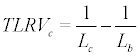(19)

Where:

TLRVc – relative investment value of general liquidity for cash.

To calculate the value of general liquidity expressed in the absolute form, it is necessary to multiply its relative value by the sum of cash funds (or by the market value of a non-cash asset, which is equivalent):

TLAVc=TLRVc X M           (20)

Where:

TLAVc – absolute investment value of general liquidity for cash funds;

M – sum of cash funds equivalent to the market value of a non-cash asset that possess a lower liquidity (as compared with cash funds).

Formulas (19) – (20) can be worded as:

General liquidity investment value = Investment value of a liquid asset – Investment value of an illiquid asset with zero yield.

Definition 2:

Relative investment value of additional liquidity for cash funds is the interest, receiving which can be readily refused by the investor for the purpose of gaining higher liquidity which is provided by cash.

Relative investment value of additional liquidity for cash funds possessing liquidity costs Lc is determined in comparison with the asset possessing the same (to cash) market value, liquidity costs Lb and yield ib, and is equal to the difference between their (Cash funds and non-cash assets possessing equivalent market value) relative investment values (or numerically to the certainty equivalent of a cash flow (CEQ) divided by a non-cash asset’s liquidity costs (Lb)):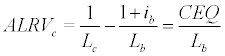(21)

Where:

ALRVc - relative investment value of additional liquidity for cash.

Absolute investment liquidity of additional liquidity for cash funds possessing liquidity costs Lc is determined in comparison with the asset possessing the same (to cash) market value, liquidity costs Lb and yield ib, and is numerically equal to the multiplication result of its relative value by the market value of the compared variables (i.e., by cash size or by the market value of a non-cash asset, which definitionally equals the value of cash):

ALAVc=ALRVc X M            (22)

Where:

ALAVc - absolute investment value of additional liquidity for cash.

Formulas (21) – (22) can be worded as:

Additional liquidity investment value = Investment value of a liquid asset – Investment value of an illiquid asset with the yield ib.

In the virtue of the given definitions, let us add the following equalities to formulas (14) – (18).

The investment value of a liquid asset is calculated as: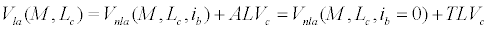(23)

Where:

Vla(M, Lc) – absolute or relative investment value of a liquid asset that possess the market value M and liquidity costs Lc;

Vnla(M, Lb, ib) – absolute and relative investment value of an illiquid asset that possess a market value M and liquidity costs Lb, and generate yield ib;

ALVc - absolute (ALAVc) or relative (ALRVc) investment value of additional liquidity for cash (the choice between the absolute and relative forms of additional liquidity values should be the same as the chosen form of the values for two other terms of the formula);

TLVc – absolute (TLAVc) and relative (TLRVc) investment value of general liquidity for cash (the choice between the absolute and relative forms of general liquidity values should be the same as the chosen form of the values for two other terms of the formula).

The investment value of an illiquid asset generating the yield ib is calculated as: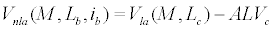(24)

Where;

Vnla(M, Lb, ib) - absolute and relative investment value of an illiquid asset that possess a market value M and liquidity costs Lb, and generate yield ib;

Vla(M, Lc) - absolute or relative investment value of a liquid asset (cash) that possess a market value M and liquidity costs Lc;

ALVc - absolute (ALAVc) or relative (ALRVc) investment value of additional liquidity for cash (the choice between the absolute and relative forms of additional liquidity values should be the same as the chosen form of the values for two other terms of the formula).

The investment value of an illiquid asset with zero yields is calculated as:

Where:

Vnla(M, Lb, ib = 0) - absolute or relative investment value of an illiquid asset that possess a market value M and liquidity costs Lb, and generate zero yields;

TLVc - absolute (TLAVc) or relative (TLRVc) investment value of general liquidity for cash (the choice between the absolute and relative forms of general liquidity values should be the same as the chosen form of the values for two other terms of the formula).

Criterion 3: Assets should be allocated in a cash form if the certainty equivalent of a cash flow (CEQ) has a positive value (as, in this case, cash possesses additional value, i.e., the investment value of liquidity). If the equivalent CEQ has a negative value, the allocation should be executed in a form of a non-cash asset (as, in this case, cash possesses negative investment value, i.e., its additional liquidity doesn’t fully compensate for the loss of profit from allocating in a non-cash asset, which generates yield ib).

The concept of “additional liquidity value” (for a cash flow) enables one to determine the profitability (or fail) of allocating cash, which possesses liquidity costs Lc, in a non-cash asset, which possesses the known yield ib and liquidity costs Lb.

The concept of “general liquidity value” (for a cash flow) enables one to determine the minimum price (equalizing interest rate), at which it becomes profitable to allocate cash possessing liquidity costs Lc in a non-cash asset possessing liquidity costs Lb and zero yields.

Based on the obtained model, we developed the algorithm for implementing the model of liquidity value estimation when choosing between investments in an asset or cash reserve:

Determine the size of temporarily surplus funds M.

Find (choose) a non-cash asset, which possesses market value equal to M.

Taking into account the parameters of the investor’s business (ib, etc.), current situation estimated by him (P1, P2, t) and characteristics of the studied non-cash asset (Lnc, inc), transform the market value of a non-cash asset M into the investment value as Vbi = M X Wb, and the market value of cash funds M into the investment value as Vci = M X Wc.

Depending on the obtained results of both the investment value of a non-cash asset and that of cash funds, make or reject the decision on investing cash into non-asset funds: if Vbi X Vci, the investment is reasonable, and if Vbi X Vci, it should be rejected.

Note 3: It should be pointed out that depending on the original interpretation of the certainty equivalent (Another possible formula for obtaining the certainty equivalent can be (ib + CEQ); in this case, the equalizing interest rate equals the CEQ), the sense of positive and negative safety margin values can change to a complete opposite. The same works for the concepts of “certainty equivalent” and “equalizing interest rate”.

Note 4: The structure of liquidity costs for cash funds Lc can differ from (6) и (7), which reflects possible situations in which the real costs of cash funds are higher than non-cash assets’ costs. Such situations deal with a higher popularity of non-cash assets as compared with cash funds. For example, the company has a lot of brick, which it doesn’t need at the moment, whilst another company is experiencing an urgent need for bricks, and it is ready to supply a lot of coal in return on better terms as compared with the cash purchase of brick on the market. Under these conditions for the given companies, the liquidity costs the non-cash assets (brick and coal) will be lower than those of cash funds.

In conclusion, we systematize the material obtained in this chapter in the form of a classification table:

Note 5: For the conditions of the aforementioned example refer Table 1, we assume that the asset’s market value = 1 billion RUB, the rate of return for a non-cash asset (bonds) (ib) = 0.12; the liquidity costs for a non-cash asset (bonds) (Lb)=1.1915; liquidity costs for cash funds (Lc) = 1.0101.

Determined type and object of value Form of expression Calculation technique Calculation of the given example
Additional liquidity investment value Relative ALRVc (Relative investment value of a liquid asset – Relative investment value of an illiquid asset possessing yield ib): 1/Lc -(1+ib)/Lb=CEQ/Lb 1/1.0101-1.12/1.1915 =0.0592/1.1915=0.05
Absolute ALAVc (Absolute investment value of cash funds – Absolute investment value of an illiquid asset possessing yield ib): Relative investment value of additional liquidity×Market value of an asset=CEQ/ Lb × Market value of an asset 0.99 billion RUB–0.94 billion RUB=0.05×1 billion RUB=0.0592/1.1915×1 billion RUB=0.05 billion RUB
Investment value of general liquidity for cash funds Relative TLRVc Relative investment value of a liquid asset – Relative investment value of an illiquid asset possessing zero yield:
(1/Lc – 1/ Lb)
0.99–1/1.1915=0.1507
Absolute TLAVc Absolute investment value of cash funds – Absolute investment value of an illiquid asset possessing zero yield:(1/Lc – 1/ Lb)×Market value of an asset 0.1507×1 billion RUB=0.151 billion RUB
Investment value of a liquid asset Relative Vla(M, Lc) Relative investment value of an illiquid asset possessing yield ib + Relative value of additional liquidity=Relative investment value of an illiquid asset possessing zero yield+Relative value of general liquidity=1/Lc 0.94+0.05=1/1.1915+0.1507=1/1.0101=0.99
Absolute Vla(M, Lc) (Sum of cash funds) / Lc or (Market value of an asset)/ Lc 1 billion RUB/1.0101=0.99 billion RUB
Investment value of an illiquid asset generating yield ib Relative Vnla(M, Lb, ib) Relative investment value of a liquid asset–Relative value of additional liquidity:(1/Lc–CEQ/Lb)=(1+ib)/Lb 0.99–0.05=0.99–0.0592/1.1915=1.12/1.1915=0.94
Absolute Vnla(M, Lb, ib) (1+ib)/Lb×(Market value of an asset) 0.94×1 billion RUB=0.94 billion RUB
Investment value of an illiquid asset possessing zero yield Relative Vnla(M, Lb, ib = 0) Relative investment value of a liquid asset–Relative value of general liquidity=1/Lb 0.99–0.1507=0.8393
Absolute Vnla(M, Lb, ib = 0) (Market value of an asset)/Lb 0.8393×1 billion RUB=0.839 billion RUB

Table 1: Classification of the investment value of liquidity and the example of its calculation with the proposed model.

If one conducts a study and obtains i=f(L) or L=g(i), it would be possible to optimize the utility function W; i.e., if one of the values i or L is defined, the value L or I is correspondingly obtained, at which the value of the function W is extreme. If i=f(L), the optimum value of liquidity costs Lopt is calculated as:

L×df(L) + f(L) = 0            (25)

Where:

df(L) – derivative of the function f(L) with respect to L.

If L = g(i), the optimum value of interest rate iopt is calculated as:

i×dg(i) + g(i) = 0            (26)

Where:

dg(i) – derivative of the function g(i) with respect to i.

#### Conclusion

The obtained results made it possible for every particular case to determine (with due regard for a known set of data) whether it is more profitable to hold either cash assets or non-cash ones.

The proposed approach leads to estimating the value of liquidity by the determination of the alternative rate of interest in the situation, when it is essential to choose between the possibility to invest temporarily surplus funds in a non-cash asset (as exampled by bonds) and a cash reserve (with a further opportunity to invest it in the main business). Nevertheless, there exist different situations, which don’t fit this scheme. For example, one can easily ask how it is possible to compare the degrees of liquidity for such absolutely different assets as gold and bread. When being intuitively clear that at different times the liquidity of bread and that of gold can change in a different manner, it stays unclear which of the two assets is more or less liquid at a certain moment. On the one hand, bread demonstrates higher liquidity, being bought on a more frequent basis; on the other hand, it is gold, which is more liquid, because of its longer shelf life. However, the author believes that the approach proposed in this study is rather reasonable, although being somewhat bounded. Undoubtedly, it is impossible to guarantee that the proposed method will allow an optimum choice to be made due to the following reasons:

• Not every possible factor is taken into account in the model;

• There is always uncertainty about the values of the model parameters P1 and P2;

• Not every market entity can adequately estimate the commensurability of the discussed alternatives, thus distorting the rates of interest formed by them (the Soros factor of imperfect understanding).

Nevertheless, it is worth mentioning that even featuring the aforementioned weaknesses, the model still enables to choose between alternatives with the definite and formalized procedure. If, in the future, one manages to synthesize more common and adequate utility functions, which reflect liquidity, taking into account not only a potential investor’s particular situation but also assets’ additional consumer properties (qualities) as well as the reflections of market entities, the theory of liquidity can be considered as completely developed.

This method can be implemented not only in case of the investment choice between cash and bonds but also in case of the choice between cash and any other asset yielding a return (e.g., immovable property) as these assets have the same characteristics including spread, fixed conversion costs, minimum required conversion time, etc.

#### Reference

Select your language of interest to view the total content in your interested language

### Article Usage

• Total views: 12282
• [From(publication date):
November-2014 - Dec 16, 2019]
• Breakdown by view type
• HTML page views : 8388Can't read the image? click here to refresh